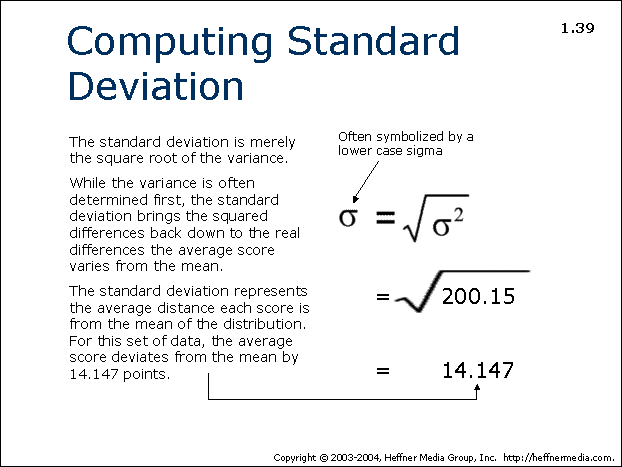# 39: Computing Standard Deviation## Computing Standard Deviation

The standard deviation is merely the square root of the variance.

While the variance is often determined first, the standard deviation brings the squared differences back down to the real differences the average score varies from the mean.

The standard deviation represents the average distance each scores is from the mean of the distribution. For this set of data, the average score deviates from the mean by 14.147 points.